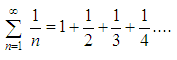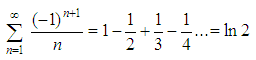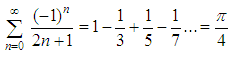# Infinite Geometric and Harmonic Series - working with numerical software

 In this article we are going to experiment on geometric and harmonic series. I suggest you read the article Sequences and Series before reading this one, to understand the basics...  An infinite geometric series is an infinite series whose successive terms have a common ratio. Such a series converges if and only if the absolute value of the common ratio is less than one.

The sum to infinity (S) of any geometric sequence in which the common ratio r is numerically less than 1 is given bywhere
a = first number of the series
r = common ratio

Example

Consider the infinite geometric series
1 – 1/2 + 1/4 – 1/8 + 1/16...
Then, a = 1 and r = -1/2

So, using the formula above the sum to infinity is
S = 1/(1-(-1/2)) = 2/3

For the series
1 – 1/3 + 1/9 – 1/27 + 1/81...
a = 1 and r = -1/3

Then, the sum to infinity is
S = 1/(1-(-1/3)) = 3/4

Let’s experiment with Matlab.

% define your first 5 n-elements
n = 0 : 4

s = (-1).^n ./ 2.^n

% find its sum
s_to_i = sum(s)

The response is:

n =     0     1     2     3     4
s =     1.0000   -0.5000    0.2500   -0.1250    0.0625
s_to_i = 0.6875

this shows that five elements are not giving us enough precision (0.6875 instead of 0.6667, as we expect). Let’s try more terms.

% use 101 elements
format long
n = 0 : 100;
s = (-1).^n ./ 2.^n;
s_to_i = sum(s)

s_to_i = 0.66666666666667, which is much better.

Obviously, we cannot directly compute a sum to infinity (it would take too long, right?) but we’re showing that using more terms we may reach an approximation as good as we need.

### Harmonic Series

A harmonic sequence is a sequence of numbers whose reciprocals form an arithmetic sequence. Then 1/2, 1/4, 1/6, 1/8... is a harmonic sequence because 2, 4, 6, 8... is an arithmetic sequence.

The harmonic series isits name derives from the concept of overtones, or harmonics in music.

Even though higher terms in the sequence are smaller in value, the series diverges. Let’s explore the series with our numerical software:

% define the first 4 terms
n = 1 : 4
seq = 1./n

% find the sum of these terms
sum(seq)

n =     1     2     3     4
seq =   1.0000    0.5000    0.3333    0.2500
ans =   2.0833

% define the first 100 terms
n = 1 : 100;
seq = 1./n;

% find the sume of thes terms
sum(seq)

ans =   5.1874

### Alternating Series

The seriesis known as the alternating harmonic series. In particular, the sum is equal to the natural logarithm of 2.

% our goal
L = log(2)

% we try 5 terms
n = 1 : 5

% define the alternating sequence
seq = (-1).^(n+1) ./ n

% get the sum
s = sum(seq)

L =     0.6931
n =     1     2     3     4     5
seq =   1.0000   -0.5000    0.3333   -0.2500    0.2000
s =     0.7833

We see that 5 terms are not enough. Let’s try 1000 terms.

% we try 1000 terms
n = 1 : 1000;

% define the alternating sequence
seq = (-1).^(n+1) ./ n;

% get the sum
s = sum(seq)

s =    0.6926

This is a closer answer, but we’re still far from log 2. More terms are needed for higher accuracy... be aware...

### Formula for pi

A related series can be derived from the Taylor series:This series starts with n = 0, and it’s known as the Leibniz formula for pi. Let’s try it in Matlab.

format long

% goal
p4 = pi/4

% we try 1000000 terms to find pi/4
n = 0 : 1000000;

% define the sequence
seq = (-1).^n ./ (2*n + 1);

% get the sum
s = sum(seq)

p4 =   0.78539816339745
s =    0.78539841339719

We see that with one-million terms in our series, we only get 6 decimals right! The series converges very slowly...

Another approach to find pi, using iterations, just for fun...

% What we’re trying to get is
% (4/1)-(4/3)+(4/5)-(4/7)...

format long

calc_pi = 0;

% Take the first 100 terms
for n = 0 : 100

% Implement the appropriate sequence
t = (-1)^n/(2*n + 1);

% Add-up the terms to try to get pi/4
calc_pi = calc_pi + t;

% Save the values to be plotted
cp(n+1) = 4*calc_pi;

end

% Plot the evolution of the algorithm
plot(cp)
title(
'Series to find pi'); grid on

% Display the last value found
cp(end)

The result for the calculated pi with 100 terms is:

ans = 3.15149340107099

This is how the series evolves. It starts with 4 and slowly tends to 3.14, but after 100 terms it has only reached 3.1514...From 'Harmonic Series ' to home

From 'Harmonic Series ' to Calculus Problems